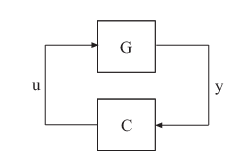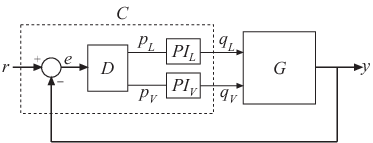# looptune

Tune fixed-structure feedback loops

## Syntax

```[G,C,gam] = looptune(G0,C0,wc) [G,C,gam] = looptune(G0,C0,wc,Req1,...,ReqN) [G,C,gam] = looptune(...,options) [G,C,gam,info] = looptune(...) ```

## Description

```[G,C,gam] = looptune(G0,C0,wc)``` tunes the feedback loopto meet the following default requirements:

• Bandwidth — Gain crossover for each loop falls in the frequency interval `wc`

• Performance — Integral action at frequencies below `wc`

• Robustness — Adequate stability margins and gain roll-off at frequencies above `wc`

The tunable `genss` model `C0` specifies the controller structure, parameters, and initial values. The model `G0` specifies the plant. `G0` can be a Numeric LTI model, or, for co-tuning the plant and controller, a tunable `genss` model. The sensor signals `y` (measurements) and actuator signals `u` (controls) define the boundary between plant and controller.

Note

For tuning Simulink® models with `looptune`, use `slTuner` (Simulink Control Design) to create an interface to your Simulink model. You can then tune the control system with `looptune` (Simulink Control Design) for `slTuner` (requires Simulink Control Design™).

```[G,C,gam] = looptune(G0,C0,wc,Req1,...,ReqN)``` tunes the feedback loop to meet additional design requirements specified in one or more tuning goal objects `Req1,...,ReqN`. Omit `wc` to use the requirements specified in `Req1,...,ReqN` instead of an explicit target crossover frequency and the default performance and robustness requirements.

```[G,C,gam] = looptune(...,options)``` specifies further options, including target gain margin, target phase margin, and computational options for the tuning algorithm.

```[G,C,gam,info] = looptune(...)``` returns a structure `info` with additional information about the tuned result. Use `info` with the `loopview` command to visualize tuning constraints and validate the tuned design.

## Input Arguments

 `G0` Numeric LTI model or tunable `genss` model representing plant in control system to tune. The plant is the portion of your control system whose outputs are sensor signals (measurements) and whose inputs are actuator signals (controls). Use `connect` to build `G0` from individual numeric or tunable components. `C0` Generalized LTI model representing controller. `C0` specifies the controller structure, parameters, and initial values. The controller is the portion of your control system that receives sensor signals (measurements) as inputs and produces actuator signals (controls) as outputs. Use Control Design Blocks and Generalized LTI models to represent tunable components of the controller. Use `connect` to build `C0` from individual numeric or tunable components. `wc` Vector specifying target crossover region `[wcmin,wcmax]`. The `looptune` command attempts to tune all loops in the control system so that the open-loop gain crosses 0 dB within the target crossover region. A scalar `wc` specifies the target crossover region `[wc/2,2*wc]`. `Req1,...,ReqN` One or more `TuningGoal` objects specifying design requirements, such as `TuningGoal.Tracking`, `TuningGoal.Gain`, or `TuningGoal.LoopShape`. `options` Set of options for `looptune` algorithm, specified using `looptuneOptions`. See `looptuneOptions` for information about the available options, including target gain margin and phase margin.

## Output Arguments

`G`

Tuned plant.

If `G0` is a Numeric LTI model, `G` is the same as `G0`.

If `G0` is a tunable `genss` model, `G` is a `genss` model with Control Design Blocks of the same number and types as `G0`. The current value of `G` is the tuned plant.

`C`

Tuned controller. `C` is a `genss` model with Control Design Blocks of the same number and types as `C0`. The current value of `C` is the tuned controller.

`gam`

Parameter indicating degree of success at meeting all tuning constraints. A value of `gam <= 1` indicates that all requirements are satisfied. `gam >> 1` indicates failure to meet at least one requirement. Use `loopview` to visualize the tuned result and identify the unsatisfied requirement.

For best results, use the `RandomStart` option in `looptuneOptions` to obtain several minimization runs. Setting `RandomStart` to an integer `N > 0` causes `looptune` to run the optimization `N` additional times, beginning from parameter values it chooses randomly. You can examine `gam` for each run to help identify an optimization result that meets your design requirements.

`info`

Data for validating tuning results, returned as a structure. To use the data in `info`, use the command `loopview(G,C,info)` to visualize tuning constraints and validate the tuned design.

`info` contains the following tuning data:

 `Di,Do` Optimal input and output scalings, returned as state-space models. The scaled plant is given by `Do\G*Di`. `Specs` Design requirements that `looptune` constructs for its call to `systune` for tuning (see Algorithms), returned as a vector of `TuningGoal` requirement objects. `Runs` Detailed information about each optimization run performed by `systune` when called by `looptune` for tuning (see Algorithms), returned as a data structure. The contents of `Runs` are the `info` output of the call to `systune`. For information about the fields of `Runs`, see the `info` output argument description on the `systune` reference page.

## Examples

Tune the control system of the following illustration, to achieve crossover between 0.1 and 1 rad/min.The 2-by-2 plant `G` is represented by:

`$G\left(s\right)=\frac{1}{75s+1}\left[\begin{array}{cc}87.8& -86.4\\ 108.2& -109.6\end{array}\right].$`

The fixed-structure controller, `C`, includes three components: the 2-by-2 decoupling matrix `D` and two PI controllers `PI_L` and `PI_V`. The signals `r`, `y`, and `e` are vector-valued signals of dimension 2.

Build a numeric model that represents the plant and a tunable model that represents the controller. Name all inputs and outputs as in the diagram, so that `looptune` knows how to interconnect the plant and controller via the control and measurement signals.

```s = tf('s'); G = 1/(75*s+1)*[87.8 -86.4; 108.2 -109.6]; G.InputName = {'qL','qV'}; G.OutputName = 'y'; D = tunableGain('Decoupler',eye(2)); D.InputName = 'e'; D.OutputName = {'pL','pV'}; PI_L = tunablePID('PI_L','pi'); PI_L.InputName = 'pL'; PI_L.OutputName = 'qL'; PI_V = tunablePID('PI_V','pi'); PI_V.InputName = 'pV'; PI_V.OutputName = 'qV'; sum1 = sumblk('e = r - y',2); C0 = connect(PI_L,PI_V,D,sum1,{'r','y'},{'qL','qV'}); wc = [0.1,1]; [G,C,gam,info] = looptune(G,C0,wc);```

`C` is the tuned controller, in this case a `genss` model with the same block types as `C0`.

You can examine the tuned result using `loopview`.

## Algorithms

`looptune` automatically converts target bandwidth, performance requirements, and additional design requirements into weighting functions that express the requirements as an H optimization problem. `looptune` then uses `systune` to optimize tunable parameters to minimize the H norm. For more information about the optimization algorithms, see .

`looptune` computes the H norm using the algorithm of  and structure-preserving eigensolvers from the SLICOT library. For more information about the SLICOT library, see http://slicot.org.

## Alternatives

For tuning Simulink models with `looptune`, see `slTuner` (Simulink Control Design) and `looptune` (Simulink Control Design) (requires Simulink Control Design).

## Compatibility Considerations

expand all

Behavior changed in R2016a

## References

 P. Apkarian and D. Noll, "Nonsmooth H-infinity Synthesis." IEEE Transactions on Automatic Control, Vol. 51, Number 1, 2006, pp. 71–86.

 Bruisma, N.A. and M. Steinbuch, "A Fast Algorithm to Compute the H-Norm of a Transfer Function Matrix," System Control Letters, 14 (1990), pp. 287-293.

## Extended Capabilities

Introduced in R2016a